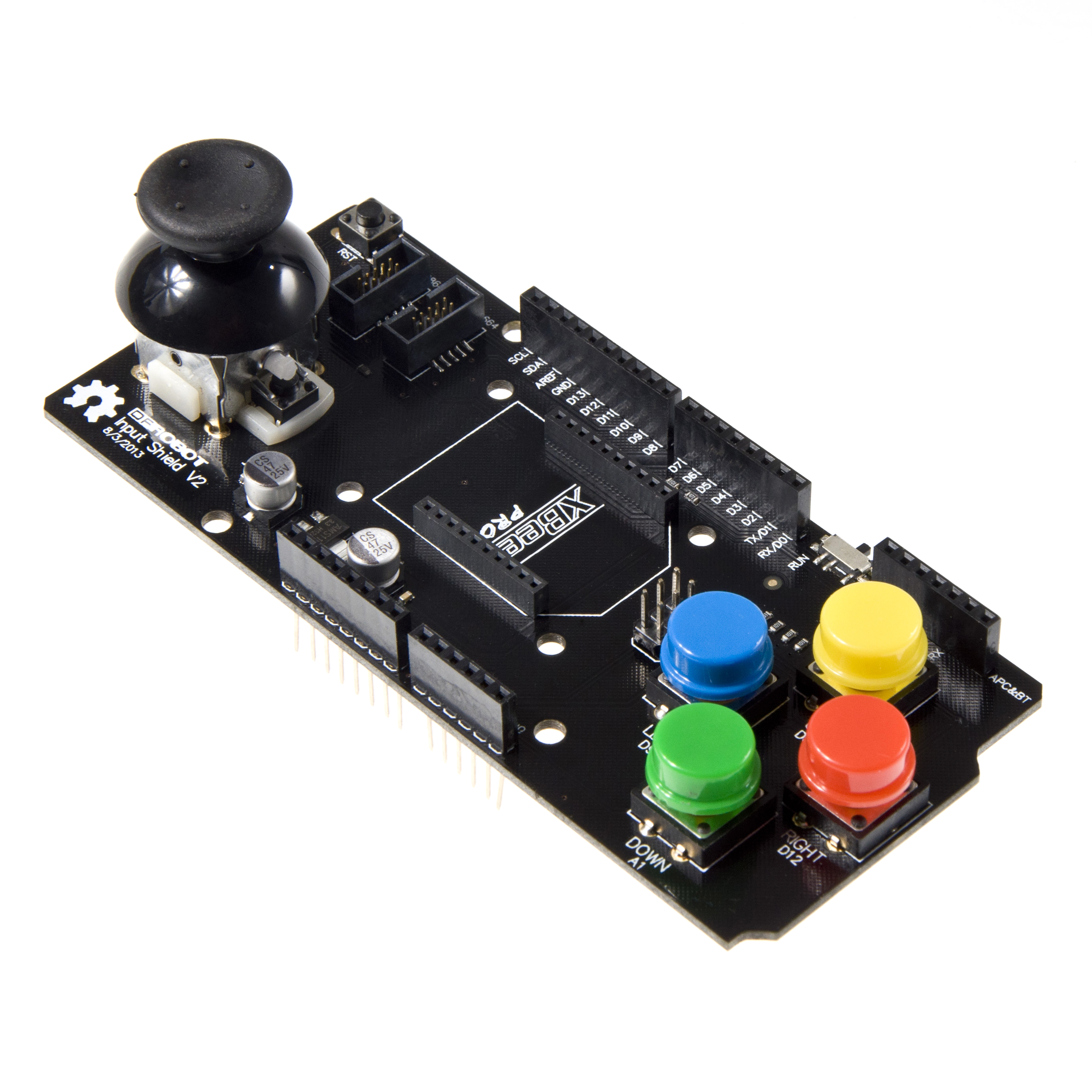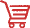# Input_Shield_For_Arduino_SKU__DFR0008-DFRobot

## IntroductionThe upgraded Arduino Input Shield includes a two axis mini joystick (with moment switch) as well as four colored push buttons(Red,Yellow,Blue,Green). The reserved APC220 Radio Data Module(SKU:TEL0005) interface and the Xbee interface is deisgned to facilitate the realization of wireless rocker button controller. The shield can be easily stacked on top of your Arduino boards. It can also connect with OLED2864 & OLED9664.

## Specification

• Dual axis mini joystick
• Connects to your Arduino using male to male pin headers
• Comes with 2 colored push buttons
• The use of imported high-quality potentiometers and the PS button rocker. The rocker output an analog signal is *to achieve vertical and horizontal control, which is attached to four keys at the same time. It also has two *great circle button caps (cap color random round keys), a rocker button and a reset button.
• The use of stack design is so that the plug can easily on Arduino Duemilanove or DFRduino Romeo.
• The reserved APC220/Bluetooth module interface is to facilitate the realization of wireless rocker button controller.
• The remaining unused ports are extended out of standby.

## Pin Allocation

Pin Function
Digital 8 UP
Analog 1 Down
Digital 9 Left
Digital 12 Right
Analog 3 X axis
Analog 2 Y axis

### Sample Code1

``````/*
*  function:   test dfr0008
*  by:         lisper (leyapin@gmail.com)
*  created:    2013-11-04
*
*/
#define up_button   8
#define down_button A1
#define left_button 9
#define right_button    12

#define stick_button    A0
#define level_stick A3
#define vertical_stick  A2

void setup () {
Serial.begin (9600);

pinMode (left_button, INPUT);
pinMode (right_button, INPUT);
pinMode (up_button, INPUT);
pinMode (down_button, INPUT);

pinMode (stick_button  , INPUT);
pinMode (level_stick   , INPUT);
pinMode (vertical_stick, INPUT);

}

void loop () {
int left_state = digitalRead (left_button);
int right_state = digitalRead (right_button);
int up_state = digitalRead (up_button);
int down_state = digitalRead (down_button);

int stick_state = digitalRead (stick_button);

int level_value = analogRead (level_stick);
int vertical_value = analogRead (vertical_stick);

Serial.print ("up=");
Serial.print (up_state);
Serial.print (" down=");
Serial.print (down_state);
Serial.print (" left=");
Serial.print (left_state);
Serial.print (" right=");
Serial.print (right_state);
Serial.print (" stick=");
Serial.print (stick_state);

Serial.print ("  vertical=");
Serial.print (vertical_value);
Serial.print (" level=");
Serial.println (level_value);

delay (500);
}``````

### Sample Code2

``````
/*
*  function:   test dfr0008
*  by:         lisper (leyapin@gmail.com)
*  created:    2013-11-04
*
*/
#include <Arduino.h>

#define up_button   8
#define down_button A1
#define left_button 9
#define right_button    12

#define stick_button A0
#define level_stick A3
#define vertical_stick A2

int vertical_value=0;   //current value
int level_value=0;      //current value
int vertical_valuep=0;  //previous value
int level_valuep=0;     //previous value

uint16_t key_delay_time = 20;   // for ispressed ()

//
void setup () {
pinMode (stick_button, INPUT);
pinMode (level_stick, INPUT);
pinMode (vertical_stick, INPUT);

pinMode (up_button, INPUT);
pinMode (down_button, INPUT);
pinMode (left_button, INPUT);
pinMode (right_button, INPUT);

Serial.begin (9600);
}

//
void loop () {

vertical_value = analogRead (vertical_stick);
level_value = analogRead (level_stick);

if (vertical_value != vertical_valuep) {
Serial.print ("vertical=");
Serial.println (vertical_value);
}
if (level_value != level_valuep) {
Serial.print ("level=");
Serial.println (level_value);
}

vertical_valuep = vertical_value;
level_valuep = level_value;

if (ispressed (up_button))
Serial.println ("up pressed");
if (ispressed (down_button))
Serial.println ("down pressed");
if (ispressed (left_button))
Serial.println ("left pressed");
if (ispressed (right_button))
Serial.println ("right pressed");
if (ispressed (stick_button))
Serial.println ("stick pressed");
delay (10);
}

//check button
boolean ispressed (uint8_t key) {
if (digitalRead (key) == 0) {
delay (key_delay_time);
if (digitalRead (key) == 0)
return true;
}
return false;
}``````

## More DocumentsGet Input Shield for Arduino from DFRobot Store or DFRobot Distributor.